# Maharashtra Board Class 10 Maths Solutions Chapter 4 Financial Planning Practice Set 4.2

## Maharashtra State Board Class 10 Maths Solutions Chapter 4 Financial Planning Practice Set 4.2

Question 1. ‘Chetana Store’ paid total GST of ₹ 1,00,500 at the time of purchase and collected GST ₹ 1,22,500 at the time of sale during 1st of July 2017 to 31st July 2017. Find the GST payable by Chetana Stores.
Output tax (Tax collected at the time of sale)
= ₹ 1,22,500
Input tax (Tax paid at the time of purchase)
= ₹ 1,00,500
ITC (Input Tax credit) = ₹ 1,00,500.
GST payable = Output tax – ITC
= 1,22,500 – 1,00,500
= ₹ 22,000
GST payable by Chetana stores is ₹ 22,000.

Question 2. Nazama is a proprietor of a firm, registered under GST. She has paid GST of ₹ 12,500 on purchase and collected ₹ 14,750 on sale. What is the amount of ITC to be claimed? What is the amount of GST payable?
Solution:
Output tax = ₹ 14,750
Input tax = ₹ 12,500
∴ ITC for Nazama = ₹ 12,500.
∴ GST payable = Output tax – ITC
= 14750 – 12500
= ₹ 2250
∴ Amount of ITC to be claimed is ₹ 12,500 and amount of GST payable is ₹ 2250.

Question 3. Amir Enterprise purchased chocolate sauce bottles and paid GST of ₹ 3800. He sold those bottles to Akbari Bros, and collected GST of ₹ 4100. Mayank Food Corner purchased these bottles from Akbari Bros, and paid GST of ₹ 4500. Find the amount of GST payable at every stage of trading and hence find payable CGST and SGST.
Solution:
For Amir Enterprise:
Output tax = ₹ 4100
Input tax = ₹ 3800
ITC for Amir enterprise = ₹ 3800.
∴ GST payable = Output tax – ITC
= 4100 – 3800
= ₹ 300
For Akbari Bros.:
Output tax = ₹ 4500
Input tax = ₹ 4100
ITC for Akbari Bros = ₹ 4100.
GST payable = Output tax – ITC
= 4500 – 4100 = ₹ 400
∴ Statement of GST payable at every stage of trading: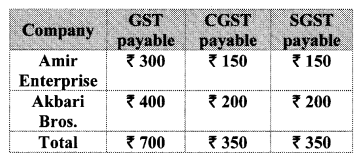Question 4. Malik Gas Agency (Chandigarh Union Territory) purchased some gas cylinders for industrial use for ₹ 24,500, and sold them to the local customers for ₹ 26,500. Find the GST to be paid at the rate of 5% and hence the CGST and UTGST to be paid for this transaction, (for Union Territories there is UTGST instead of SGST.)
Solution:
For Malik Gas Agency:
Output tax = 5% of 26500
= $$\frac { 5 }{ 100 }$$ × 26500
= ₹ 1325
Input tax = 5% of 24500
= $$\frac { 5 }{ 100 }$$ × 24500
= ₹ 1225
ITC for Malik Gas Agency = ₹ 1225.
∴ GST payable = Output tax – ITC
= 1325 – 1225
= ₹ 100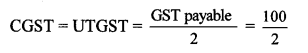∴ CGST = UTGST = ₹ 50
∴ The GST to be paid at the rate of 5% is ₹ 100 and hence, CGST and UTGST paid for the transaction is ₹ 50 each.

Question 5.
M/s Beauty Products paid 18% GST on cosmetics worth ₹ 6000 and sold to a customer for ₹ 10,000. What are the amounts of CGST and SGST shown in the tax invoice issued?
Solution:
Output tax = 18% of 10,000
= $$\frac { 18 }{ 100 }$$ × 10,000
= ₹ 1800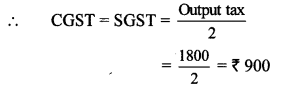∴ Amount of CGST and SGST shown in the tax invoice issued is ₹ 900 each.

Question 6.
Prepare Business to Consumer (B2C) tax invoice using given information. Write the name of the supplier, address, state, Date, Invoice number, GSTIN etc. as per your choice.
Supplier: M/s ______ Address _______ State _______ Date _______ Invoice No. _______ GSTIN _______
Particulars
Rate of Mobile Battery ₹ 200 Rate of GST 12% HSN 8507 1 PC
Rate of Headphone ₹750 Rate of GST 18% HSN 8518 1 Pc
Solution:
Rate of Mobile Battery = ₹200
CGST = 6% of 200
= $$\frac { 6 }{ 100 }$$ × 200
= ₹ 12
∴ CGST = SGST = ₹ 12

Rate of Headphone = ₹ 750
COST = 9% of 750
= $$\frac { 9 }{ 100 }$$ × 750
= ₹ 67.5
∴ CGST = SGST = ₹ 67.5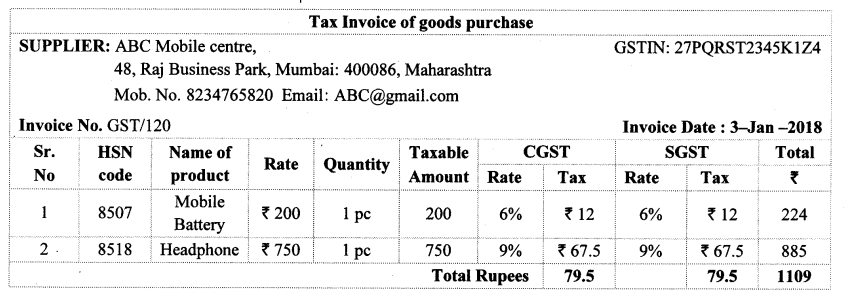Question 7.
Prepare Business to Business (B2B) Tax Invoice as per the details given below, name of the supplier, address, Date etc. as per your choice.
Supplier – Name, Address, State, GSTIN, Invoice No., Date
Recipient – Name, Address, State, GSTIN,
Items:
i. Pencil boxes 100, HSN – 3924, Rate – ₹ 20, GST 12%
ii. Jigsaw Puzzles 50, HSN 9503, Rate – ₹ 100 GST 12%.
Solution:
Cost of 100 Pencil boxes
= 20 × 1oo
= ₹ 2000
CGST = 6% of 2000
= $$\frac { 6 }{ 100 }$$ × 2000
= ₹ 120
∴ CGST = SGST = ₹ 120

Cost of 50 Jigsaw Puzzles = 100 × 50
= ₹ 5000
CGST = 6% of 5000
= $$\frac { 6 }{ 100 }$$ × 5000
= ₹ 300
CGST – SGST = ₹ 300Question 1.
Suppose a manufacturer sold a cycle for a taxable value of ₹ 4000 to the wholesaler. Wholesaler sold it to the retailer for ₹ 4800 (taxable value). Retailer sold it to a customer for ₹ 5200 (taxable value). Rate of GST is 12%. Complete the following activity to find the payable CGST and SGST at each stage of trading. (Textbook pg. no. 92)
Solution: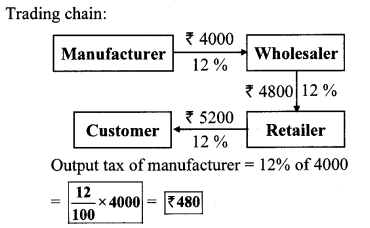GST payable by manufacturer = ₹ 480
Output tax of wholesaler
= 12% of 4800 = $$\frac { 12 }{ 100 }$$ × 4800 = ₹ 576
∴ GST payable by wholesaler
= Output tax – Input tax
= 576 – 480
= ₹ 96
Output tax of retailer = 12% of 5200Question 2. Suppose in the month of July the output tax of a trader is equal to the input tax, then what is his payable GST?(Textbook pg. no. 93)